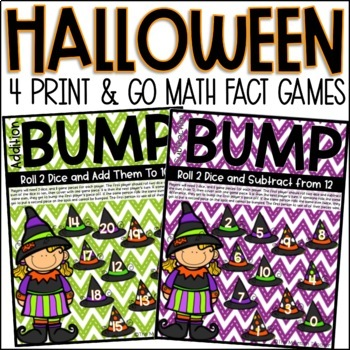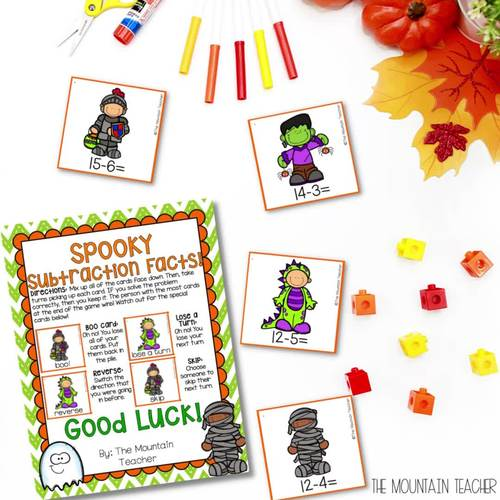# Halloween Math Activities - Addition and Subtraction BUMP Math Games

Rated 5 out of 5, based on 1 reviews
1 Rating;
K - 3rd
Subjects
Resource Type
Standards
Formats Included
• PDF
Pages
19 pages
Report this resource to TPT

### Description

Looking for the best Halloween math activities? Students LOVE these fun BUMP Halloween math games. Students will practice adding and subtracting to 20 with these four engaging Halloween math activities including 4 different games.

Halloween Math Activities Included:

• Addition to 20 BUMP Game
• Subtraction under 20 BUMP Game
• Addition to 20 Boo Game
• Subtraction to 20 Boo Game

Math Skills:

These Halloween math games are great for any primary grade to help students begin to fluently solve adding and subtracting to 20 problems. There are 2 core games included, which have an addition version and subtraction version. The games are Bump and Boo!

These Halloween math activities are best fitted for kindergarten, 1st, 2nd or 3rd graders. Students will need to know how to add and subtract to 20.

Bump Directions:

Players will need 2 dice, and 8 game pieces for each player. The first player should roll two dice and add the sum or difference of the dice to a certain number. Cover the sum with one game piece. It is then the next persons turn. If someone rolls the same sum, they get to bump the first player’s piece off. If someone rolls the same sum twice, they get to put a second piece on the space and cannot be bumped. The first person to use all 8 of their pieces wins.

Boo Directions:

Mix up all of the cards face down. Then, take turns picking up each card. If you solve the problem correctly, then you keep it. The person with the most cards at the end of the game wins! Watch out for the special cards Boo, Reverse, Skip, Lose a Turn.

Teacher Prep:

Either print, laminate and cut these Halloween math activities for use year after year OR print and let students use without laminating.

When to Use in Your Classroom:

• Halloween Party Day
• Math Center Time
• Independent Math Work
• Early Finisher Activity
• Small Group Review
• RTI or Enrichment
• Daily Math Warm Up

Interested in other Halloween activities for your first, second or third graders? Check these student favorites out below!

You can also connect with me for first access to my resources, giveaways and FREEBIES via: Email | Website | Instagram | Facebook | Teachers Pay Teachers

Total Pages
19 pages
Does not apply
Teaching Duration
1 month
Report this resource to TPT
Reported resources will be reviewed by our team. Report this resource to let us know if this resource violates TPT’s content guidelines.

### Standards

to see state-specific standards (only available in the US).
Add within 100, including adding a two-digit number and a one-digit number, and adding a two-digit number and a multiple of 10, using concrete models or drawings and strategies based on place value, properties of operations, and/or the relationship between addition and subtraction; relate the strategy to a written method and explain the reasoning used. Understand that in adding two-digit numbers, one adds tens and tens, ones and ones; and sometimes it is necessary to compose a ten.
Fluently add and subtract within 1000 using strategies and algorithms based on place value, properties of operations, and/or the relationship between addition and subtraction.
Solve addition and subtraction word problems, and add and subtract within 10, e.g., by using objects or drawings to represent the problem.
Fluently add and subtract within 5.
Add and subtract within 20, demonstrating fluency for addition and subtraction within 10. Use strategies such as counting on; making ten (e.g., 8 + 6 = 8 + 2 + 4 = 10 + 4 = 14); decomposing a number leading to a ten (e.g., 13 - 4 = 13 - 3 - 1 = 10 - 1 = 9); using the relationship between addition and subtraction (e.g., knowing that 8 + 4 = 12, one knows 12 - 8 = 4); and creating equivalent but easier or known sums (e.g., adding 6 + 7 by creating the known equivalent 6 + 6 + 1 = 12 + 1 = 13).